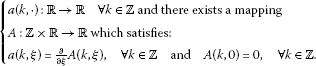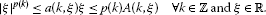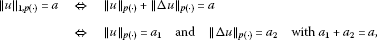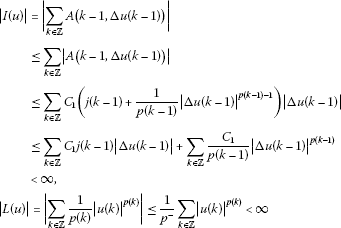Theory and Modern Applications

# Weak homoclinic solutions of anisotropic difference equation with variable exponents

## Abstract

In this paper, we prove the existence of homoclinic solutions for a family of anisotropic difference equations. The proof of the main result is based on a minimization method and a discrete Hölder type inequality.

MSC:47A75, 35B38, 35P30, 34L05, 34L30.

## 1 Introduction

In this paper, we study the following nonlinear discrete anisotropic problem:

$\left\{\begin{array}{cc}-\mathrm{\Delta }\left(a\left(k-1,\mathrm{\Delta }u\left(k-1\right)\right)\right)+|u\left(k\right){|}^{p\left(k\right)-2}u\left(k\right)=f\left(k\right),\hfill & k\in \mathbb{Z},\hfill \\ {lim}_{|k|\to \mathrm{\infty }}u\left(k\right)=0,\hfill \end{array}$
(1.1)

where $\mathrm{\Delta }u\left(k\right)=u\left(k+1\right)-u\left(k\right)$ is the forward difference operator.

The problem (1.1) is a class of partial difference equations which usually describe the evolution of certain phenomena over the course of time. Elementary but relevant examples of partial difference equations are concerned with heat diffusion, heat control, temperature distribution, population growth, cellular neural networks, etc. (see ). Our interest for problems of the type (1.1) is motivated by major applications of differential and difference operators to various applied fields such as electrorheological (smart) fluids, space technology, robotics, image processing, etc. On the other hand, they are strongly motivated by their applicability to mathematical physics and biology.

The goal of the present paper is to establish the existence of homoclinic solutions for the problem (1.1). In the theory of differential equations, a trajectory $x\left(t\right)$, which is asymptotic to a constant as $|t|\to \mathrm{\infty }$, is called a doubly asymptotic or homoclinic orbit. The notion of a homoclinic orbit was introduced by Poincaré  for continuous Hamiltonian systems. Since we are seeking for solutions u of the problem (1.1) satisfying ${lim}_{|k|\to \mathrm{\infty }}u\left(k\right)=0$, then according to the notion of a homoclinic orbit by Poincaré , we are interested in finding homoclinic solutions for the problem (1.1). We remember that boundary value problems involving difference operators with constant exponents have been intensively studied in the last decade (see  for details). The existence of homoclinic solutions where studied in particular by the authors in . The variable exponent cases were studied by some authors in  and the references therein. Other very recent applications of variable exponent equations are presented in . The study of such kind of problems can be placed at the interface of certain mathematical fields such as nonlinear differential equations and numerical analysis.

The variational approach to the study of homoclinic solutions in the context of variable exponent was firstly done by Mihailescu, Radulescu and Tersian in . They studied the following problem:

(1.2)

where ${\mathrm{\Delta }}_{p\left(\cdot \right)}^{2}$ stands for the $p\left(\cdot \right)$-Laplace difference operator, that is,

${\mathrm{\Delta }}_{p\left(k-1\right)}^{2}u\left(k-1\right)=|\mathrm{\Delta }u\left(k\right){|}^{p\left(k\right)-2}\mathrm{\Delta }u\left(k\right)-|\mathrm{\Delta }u\left(k-1\right){|}^{p\left(k-1\right)-2}\mathrm{\Delta }u\left(k-1\right),$

for each $k\in \mathbb{Z}$.

In this paper, we use the minimization technique to get the existence of homoclinic solutions of (1.1).

The paper is organized as follows. In Section 2, we define the functional spaces and prove some of their useful properties, and finally, in Section 3, we prove the existence of homoclinic solutions of (1.1).

## 2 Auxiliary results

We will use the following notations from now on:

${p}^{+}=\underset{k\in \mathbb{Z}}{sup}p\left(k\right)\phantom{\rule{1em}{0ex}}\text{and}\phantom{\rule{1em}{0ex}}{p}^{-}=\underset{k\in \mathbb{Z}}{inf}p\left(k\right).$

For the data f and a, we assume the following:(2.1)(2.2)

There exists a positive constant ${C}_{1}$ such that

$|a\left(k,\xi \right)|\le {C}_{1}\left(j\left(k\right)+|\xi {|}^{p\left(k\right)-1}\right),$
(2.3)

for all $k\in \mathbb{Z}$ and $\xi \in \mathbb{R}$, where $j\in {l}^{{p}^{\prime }\left(\cdot \right)}$ (a space to be defined later) with $\frac{1}{p\left(k\right)}+\frac{1}{{p}^{\prime }\left(k\right)}=1$.

$f\in {l}^{{p}^{\prime }\left(\cdot \right)},$
(2.4)

with $\frac{1}{p\left(k\right)}+\frac{1}{{p}^{\prime }\left(k\right)}=1$, for all $k\in \mathbb{Z}$.

(2.5)

We now introduce the spaces:

${l}^{p\left(\cdot \right)}=\left\{u:\mathbb{Z}\to \mathbb{R};{\rho }_{p\left(\cdot \right)}\left(u\right):=\sum _{k\in \mathbb{Z}}|u\left(k\right){|}^{p\left(k\right)}<\mathrm{\infty }\right\}$

and

On ${l}^{p\left(\cdot \right)}$, we introduce the Luxemburg norm

${\parallel u\parallel }_{p\left(\cdot \right)}:=inf\left\{\lambda >0;\sum _{k\in \mathbb{Z}}|\frac{u\left(k\right)}{\lambda }{|}^{p\left(k\right)}\le 1\right\}.$

Then

$\begin{array}{rcl}{\parallel u\parallel }_{1,p\left(\cdot \right)}& :=& inf\left\{\lambda >0;\sum _{k\in \mathbb{Z}}|\frac{u\left(k\right)}{\lambda }{|}^{p\left(k\right)}+\sum _{k\in \mathbb{Z}}|\frac{\mathrm{\Delta }u\left(k\right)}{\lambda }{|}^{p\left(k\right)}\le 1\right\}\\ =& {\parallel u\parallel }_{p\left(\cdot \right)}+{\parallel \mathrm{\Delta }u\parallel }_{p\left(\cdot \right)}\end{array}$

is a norm on the space ${\mathcal{W}}^{1,p\left(\cdot \right)}$.

Remark 2.1 If $u\in {l}^{p\left(\cdot \right)}$, then ${lim}_{|k|\to +\mathrm{\infty }}u\left(k\right)=0$. Indeed, if $u\in {l}^{p\left(\cdot \right)}$, then ${\sum }_{k\in \mathbb{Z}}|u\left(k\right){|}^{p\left(k\right)}<\mathrm{\infty }$. Let

$\sum _{k\in \mathbb{Z}}|u\left(k\right){|}^{p\left(k\right)}=\sum _{k\in {S}_{1}}|u\left(k\right){|}^{p\left(k\right)}+\sum _{k\in {S}_{2}}|u\left(k\right){|}^{p\left(k\right)},$

where

${S}_{1}=\left\{k\in \mathbb{Z};|u\left(k\right)|<1\right\}$

and

${S}_{2}=\left\{k\in \mathbb{Z};|u\left(k\right)|\ge 1\right\}.$

${S}_{2}$ is necessarily a finite set and $|u\left(k\right)|<\mathrm{\infty }$ for any $k\in {S}_{2}$ since $u\in {l}^{p\left(\cdot \right)}$.

We also have that ${\sum }_{k\in {S}_{1}}|u\left(k\right){|}^{{p}^{+}}\le {\sum }_{k\in \mathbb{Z}}|u\left(k\right){|}^{p\left(k\right)}$, then ${\sum }_{k\in {S}_{1}}|u\left(k\right){|}^{{p}^{+}}<\mathrm{\infty }$. As ${S}_{2}$ is a finite set, then ${\sum }_{k\in {S}_{2}}|u\left(k\right){|}^{{p}^{+}}<\mathrm{\infty }$, which implies that

$\sum _{k\in \mathbb{Z}}|u\left(k\right){|}^{{p}^{+}}<\mathrm{\infty }.$

Thus,

$\underset{|k|\to +\mathrm{\infty }}{lim}u\left(k\right)=0.$

Proposition 2.1 Under condition (2.5), ${\rho }_{p\left(\cdot \right)}$ satisfies:

1. (a)

${\rho }_{p\left(\cdot \right)}\left(u+v\right)\le {2}^{p+}\left({\rho }_{p\left(\cdot \right)}\left(u\right)+{\rho }_{p\left(\cdot \right)}\left(v\right)\right)$; $\mathrm{\forall }u,v\in {l}^{p\left(\cdot \right)}$.

2. (b)

For $u\in {l}^{p\left(\cdot \right)}$, if $\lambda >1$, we have

${\rho }_{p\left(\cdot \right)}\left(u\right)\le \lambda {\rho }_{p\left(\cdot \right)}\left(u\right)\le {\lambda }^{{p}^{-}}{\rho }_{p\left(\cdot \right)}\left(u\right)\le {\rho }_{p\left(\cdot \right)}\left(\lambda u\right)\le {\lambda }^{{p}^{+}}{\rho }_{p\left(\cdot \right)}\left(u\right),$

and if $0<\lambda <1$, we have

${\lambda }^{{p}^{+}}{\rho }_{p\left(\cdot \right)}\left(u\right)\le {\rho }_{p\left(\cdot \right)}\left(\lambda u\right)\le {\lambda }^{{p}^{-}}{\rho }_{p\left(\cdot \right)}\left(u\right)\le \lambda {\rho }_{p\left(\cdot \right)}\left(u\right)\le {\rho }_{p\left(\cdot \right)}\left(u\right).$
1. (c)

For every fixed $u\in {l}^{p\left(\cdot \right)}\setminus \left\{0\right\}$, ${\rho }_{p\left(\cdot \right)}\left(\lambda u\right)$ is a continuous convex even function in λ, and it increases strictly when $\lambda \in \left[0,\mathrm{\infty }\right)$.

Proof (a) Let $u,v\in {l}^{p\left(\cdot \right)}$, we have

$\begin{array}{rcl}{\rho }_{p\left(\cdot \right)}\left(u+v\right)& =& \sum _{k\in \mathbb{Z}}|u\left(k\right)+v\left(k\right){|}^{p\left(k\right)}\\ =& \sum _{k\in \mathbb{Z}}{2}^{p\left(k\right)}|\frac{1}{2}u\left(k\right)+\frac{1}{2}v\left(k\right){|}^{p\left(k\right)}\\ \le & \sum _{k\in \mathbb{Z}}{2}^{p\left(k\right)}\left[\frac{1}{2}|u\left(k\right){|}^{p\left(k\right)}+\frac{1}{2}|v\left(k\right){|}^{p\left(k\right)}\right]\\ \le & {2}^{{p}^{+}-1}\left({\rho }_{p\left(\cdot \right)}\left(u\right)+{\rho }_{p\left(\cdot \right)}\left(v\right)\right)\\ \le & {2}^{{p}^{+}}\left({\rho }_{p\left(\cdot \right)}\left(u\right)+{\rho }_{p\left(\cdot \right)}\left(v\right)\right).\end{array}$
1. (b)

For $u\in {l}^{p\left(\cdot \right)}$, if $\lambda >1$, we have

$\begin{array}{rcl}{\rho }_{p\left(\cdot \right)}\left(\lambda u\right)& =& \sum _{k\in \mathbb{Z}}|\lambda u\left(k\right){|}^{p\left(k\right)}\\ =& \sum _{k\in \mathbb{Z}}{\lambda }^{p\left(k\right)}|u\left(k\right){|}^{p\left(k\right)}\\ \ge & {\lambda }^{{p}^{-}}\sum _{k\in \mathbb{Z}}|u\left(k\right){|}^{p\left(k\right)}={\lambda }^{{p}^{-}}{\rho }_{p\left(\cdot \right)}\left(u\right).\end{array}$

We also have ${\rho }_{p\left(\cdot \right)}\left(\lambda u\right)={\sum }_{k\in \mathbb{Z}}{\lambda }^{p\left(k\right)}|u\left(k\right){|}^{p\left(k\right)}\le {\lambda }^{{p}^{+}}{\sum }_{k\in \mathbb{Z}}|u\left(k\right){|}^{p\left(k\right)}={\lambda }^{{p}^{+}}{\rho }_{p\left(\cdot \right)}\left(u\right)$.

If $0<\lambda <1$, we have

$\begin{array}{rcl}{\rho }_{p\left(\cdot \right)}\left(\lambda u\right)& =& \sum _{k\in \mathbb{Z}}|\lambda u\left(k\right){|}^{p\left(k\right)}\\ =& \sum _{k\in \mathbb{Z}}{\lambda }^{p\left(k\right)}|u\left(k\right){|}^{p\left(k\right)}\\ \ge & {\lambda }^{{p}^{+}}\sum _{k\in \mathbb{Z}}|u\left(k\right){|}^{p\left(k\right)}={\lambda }^{{p}^{+}}{\rho }_{p\left(\cdot \right)}\left(u\right).\end{array}$

We also have ${\rho }_{p\left(\cdot \right)}\left(\lambda u\right)={\sum }_{k\in \mathbb{Z}}{\lambda }^{p\left(k\right)}|u\left(k\right){|}^{p\left(k\right)}\le {\lambda }^{{p}^{-}}{\sum }_{k\in \mathbb{Z}}|u\left(k\right){|}^{p\left(k\right)}={\lambda }^{{p}^{-}}{\rho }_{p\left(\cdot \right)}\left(u\right)$.

1. (c)

For every fixed $u\in {l}^{p\left(\cdot \right)}\setminus \left\{0\right\}$ and $\alpha \in \left(0,1\right)$, we have $\mathrm{\forall }v\in {l}^{p\left(\cdot \right)}$

$\begin{array}{rcl}{\rho }_{p\left(\cdot \right)}\left(\alpha u+\left(1-\alpha \right)v\right)& \le & \sum _{k\in \mathbb{Z}}\left[|\alpha u\left(k\right){|}^{p\left(k\right)}+|\left(1-\alpha \right)v\left(k\right){|}^{p\left(k\right)}\right]\\ \le & \sum _{k\in \mathbb{Z}}\left[{\alpha }^{p\left(k\right)}|u\left(k\right){|}^{p\left(k\right)}+{\left(1-\alpha \right)}^{p\left(k\right)}|v\left(k\right){|}^{p\left(k\right)}\right]\\ \le & \sum _{k\in \mathbb{Z}}\left[\alpha |u\left(k\right){|}^{p\left(k\right)}+\left(1-\alpha \right)|v\left(k\right){|}^{p\left(k\right)}\right]\\ \le & \alpha {\rho }_{p\left(\cdot \right)}\left(u\right)+\left(1-\alpha \right){\rho }_{p\left(\cdot \right)}\left(v\right).\end{array}$

This proves that ${\rho }_{p\left(\cdot \right)}\left(u\right)$ is convex.

Let ${\lambda }_{1},{\lambda }_{2}\ge 0$ such that ${\lambda }_{1}<{\lambda }_{2}$. We have

$\begin{array}{rcl}{\rho }_{p\left(\cdot \right)}\left({\lambda }_{1}u\right)& =& \sum _{k\in \mathbb{Z}}|{\lambda }_{1}u\left(k\right){|}^{p\left(k\right)}\\ =& \sum _{k\in \mathbb{Z}}{\lambda }_{1}^{p\left(k\right)}|u\left(k\right){|}^{p\left(k\right)}\\ <& \sum _{k\in \mathbb{Z}}{\lambda }_{2}^{p\left(k\right)}|u\left(k\right){|}^{p\left(k\right)}={\rho }_{p\left(\cdot \right)}\left({\lambda }_{2}u\right).\end{array}$

Thus, for every fixed $u\in {l}^{p\left(\cdot \right)}\setminus \left\{0\right\}$, ${\rho }_{p\left(\cdot \right)}\left(\lambda u\right)$ increases strictly when $\lambda \in \left[0,+\mathrm{\infty }\right)$.

For the continuity of ${\rho }_{p\left(\cdot \right)}\left(\lambda u\right)$, let ${\left({\lambda }_{n}\right)}_{n\in \mathbb{N}}$ be a real sequence such that ${\lambda }_{n}\to \lambda$ as $n\to +\mathrm{\infty }$. We denote ${g}_{n,k}=|{\lambda }_{n}u\left(k\right){|}^{p\left(k\right)}$. We have

$\sum _{k\in \mathbb{Z}}|{g}_{n,k}|\le |{\lambda }_{n}{|}^{\beta }\sum _{k\in \mathbb{Z}}|u\left(k\right)|<+\mathrm{\infty },$

where

Then,

$\underset{n\to +\mathrm{\infty }}{lim}\left(\sum _{k\in \mathbb{Z}}|{\lambda }_{n}u{|}^{p\left(k\right)}\right)=\sum _{k\in \mathbb{Z}}\underset{n\to +\mathrm{\infty }}{lim}|{\lambda }_{n}u{|}^{p\left(k\right)}=\sum _{k\in \mathbb{Z}}|\lambda u{|}^{p\left(k\right)}.$

Therefore, we have the continuity of ${\rho }_{p\left(\cdot \right)}$. □

Proposition 2.2 Let $u\in {l}^{p\left(\cdot \right)}\setminus \left\{0\right\}$, then ${\parallel u\parallel }_{p\left(\cdot \right)}=a$ if and only if ${\rho }_{p\left(\cdot \right)}\left(\frac{u}{a}\right)=1$.

Proof Let us denote $A=\left\{\lambda >0;{\rho }_{p\left(\cdot \right)}\left(\frac{u}{\lambda }\right)\le 1\right\}$.

Case 1: ${\parallel u\parallel }_{p\left(\cdot \right)}=a$. Then, there exists a sequence ${\left({\lambda }_{n}\right)}_{n\in \mathbb{N}}\subset A$ such that .

Therefore, as ${\rho }_{p\left(\cdot \right)}\left(\frac{u}{{\lambda }_{n}}\right)\le 1$ for all $n\in \mathbb{N}$ and ${\rho }_{p\left(\cdot \right)}\left(\lambda u\right)$ is continuous with respect to λ, then we get ${\rho }_{p\left(\cdot \right)}\left(\frac{u}{a}\right)\le 1$.

Suppose now that ${\rho }_{p\left(\cdot \right)}\left(\frac{u}{a}\right)<1$. As $a>0$, then there exists $\alpha >0$ such that $0<\alpha . Then

${\rho }_{p\left(\cdot \right)}\left(\frac{u}{\alpha }\right)={\rho }_{p\left(\cdot \right)}\left(\frac{u}{a}×\frac{a}{\alpha }\right)\le {\left(\frac{a}{\alpha }\right)}^{{p}^{+}}{\rho }_{p\left(\cdot \right)}\left(\frac{u}{\alpha }\right).$

Solving the inequality ${\left(\frac{a}{\alpha }\right)}^{{p}^{+}}{\rho }_{p\left(\cdot \right)}\left(\frac{u}{\alpha }\right)\le 1$, we get

$\alpha \ge \frac{a}{{\left(\frac{1}{{\rho }_{p\left(\cdot \right)}\left(\frac{u}{a}\right)}\right)}^{\frac{1}{{p}^{+}}}}.$

Since

$0<\frac{a}{{\left(\frac{1}{{\rho }_{p\left(\cdot \right)}\left(\frac{u}{a}\right)}\right)}^{\frac{1}{{p}^{+}}}}

then there exists $0<\alpha such that ${\rho }_{p\left(\cdot \right)}\left(\frac{u}{\alpha }\right)\le 1$, which is a contradiction.

Thus,

${\rho }_{p\left(\cdot \right)}\left(\frac{u}{a}\right)=1.$

Case 2: ${\rho }_{p\left(\cdot \right)}\left(\frac{u}{a}\right)=1$. Then, $a\in A$ and ${\parallel u\parallel }_{p\left(\cdot \right)}\le a$. Let ${\left({\lambda }_{n}\right)}_{n\in \mathbb{N}}\subset A$ be a sequence such that ${\lambda }_{n}↓{\parallel u\parallel }_{p\left(\cdot \right)}$.

We suppose that ${\parallel u\parallel }_{p\left(\cdot \right)}, then

$1={\rho }_{p\left(\cdot \right)}\left(\frac{u}{a}\right)={\rho }_{p\left(\cdot \right)}\left(\frac{u}{{\parallel u\parallel }_{p\left(\cdot \right)}}×\frac{{\parallel u\parallel }_{p\left(\cdot \right)}}{a}\right)\le {\left(\frac{{\parallel u\parallel }_{p\left(\cdot \right)}}{a}\right)}^{{p}^{-}}{\rho }_{p\left(\cdot \right)}\left(\frac{u}{{\parallel u\parallel }_{p\left(\cdot \right)}}\right).$

So

${\rho }_{p\left(\cdot \right)}\left(\frac{u}{{\parallel u\parallel }_{p\left(\cdot \right)}}\right)\ge \frac{1}{{\left(\frac{{\parallel u\parallel }_{p\left(\cdot \right)}}{a}\right)}^{{p}^{-}}},$

which implies that

${\rho }_{p\left(\cdot \right)}\left(\frac{u}{{\parallel u\parallel }_{p\left(\cdot \right)}}\right)>1.$

Therefore,

$1<{\rho }_{p\left(\cdot \right)}\left(\frac{u}{{\parallel u\parallel }_{p\left(\cdot \right)}}\right)=\underset{n\to +\mathrm{\infty }}{lim}{\rho }_{p\left(\cdot \right)}\left(\frac{u}{{\lambda }_{n}}\right)\le 1,$

${\parallel u\parallel }_{p\left(\cdot \right)}=a.$

□

Proposition 2.3 If $u\in {l}^{p\left(\cdot \right)}$ and ${p}^{+}<+\mathrm{\infty }$, then the following properties hold:

1. (1)

${\parallel u\parallel }_{p\left(\cdot \right)}<1\phantom{\rule{0.25em}{0ex}}\left(=1;>1\right)⇔{\rho }_{p\left(\cdot \right)}\left(u\right)<1\phantom{\rule{0.25em}{0ex}}\left(=1;>1\right)$;

2. (2)

${\parallel u\parallel }_{p\left(\cdot \right)}>1⇒{\parallel u\parallel }_{p\left(\cdot \right)}^{{p}^{-}}\le {\rho }_{p\left(\cdot \right)}\left(u\right)\le {\parallel u\parallel }_{p\left(\cdot \right)}^{p+}$;

3. (3)

${\parallel u\parallel }_{p\left(\cdot \right)}<1⇒{\parallel u\parallel }_{p\left(\cdot \right)}^{{p}^{+}}\le {\rho }_{p\left(\cdot \right)}\left(u\right)\le {\parallel u\parallel }_{p\left(\cdot \right)}^{p-}$;

4. (4)

${\parallel {u}_{n}\parallel }_{p\left(\cdot \right)}\to 0⇔{\rho }_{p\left(\cdot \right)}\left({u}_{n}\right)\to 0$ as $n\to +\mathrm{\infty }$.

Proof (1) ${\parallel u\parallel }_{p\left(\cdot \right)}<1\phantom{\rule{0.25em}{0ex}}\left(=1;>1\right)⇔{\rho }_{p\left(\cdot \right)}\left(u\right)<1\phantom{\rule{0.25em}{0ex}}\left(=1;>1\right)$.

Case 1. ${\parallel u\parallel }_{p\left(\cdot \right)}=1⇔{\rho }_{p\left(\cdot \right)}\left(u\right)=1$ is proven using Proposition 2.2.

Case 2. ${\parallel u\parallel }_{p\left(\cdot \right)}<1⇔{\rho }_{p\left(\cdot \right)}\left(u\right)<1$. Suppose that ${\parallel u\parallel }_{p\left(\cdot \right)}<1$, then

${\rho }_{p\left(\cdot \right)}\left(u\right)={\rho }_{p\left(\cdot \right)}\left(\frac{u}{{\parallel u\parallel }_{p\left(\cdot \right)}}×{\parallel u\parallel }_{p\left(\cdot \right)}\right)\le {\parallel u\parallel }_{p\left(\cdot \right)}^{{p}^{-}}{\rho }_{p\left(\cdot \right)}\left(\frac{u}{{\parallel u\parallel }_{p\left(\cdot \right)}}\right)\le {\parallel u\parallel }_{p\left(\cdot \right)}^{{p}^{-}}<1.$

Conversely, taking ${\rho }_{p\left(\cdot \right)}\left(u\right)<1$ and supposing that ${\parallel u\parallel }_{p\left(\cdot \right)}\ge 1$, then

$1>{\rho }_{p\left(\cdot \right)}\left(u\right)={\rho }_{p\left(\cdot \right)}\left(\frac{u}{{\parallel u\parallel }_{p\left(\cdot \right)}}×{\parallel u\parallel }_{p\left(\cdot \right)}\right)\ge {\parallel u\parallel }_{p\left(\cdot \right)}^{{p}^{-}}{\rho }_{p\left(\cdot \right)}\left(\frac{u}{{\parallel u\parallel }_{p\left(\cdot \right)}}\right)={\parallel u\parallel }_{p\left(\cdot \right)}^{{p}^{-}},$

which is a contradiction. Therefore, ${\parallel u\parallel }_{p\left(\cdot \right)}<1$.

Case 3. ${\parallel u\parallel }_{p\left(\cdot \right)}>1⇔{\rho }_{p\left(\cdot \right)}\left(u\right)>1$. Suppose that ${\parallel u\parallel }_{p\left(\cdot \right)}>1$, then

${\rho }_{p\left(\cdot \right)}\left(u\right)={\rho }_{p\left(\cdot \right)}\left(\frac{u}{{\parallel u\parallel }_{p\left(\cdot \right)}}×{\parallel u\parallel }_{p\left(\cdot \right)}\right)\ge {\parallel u\parallel }_{p\left(\cdot \right)}^{{p}^{-}}{\rho }_{p\left(\cdot \right)}\left(\frac{u}{{\parallel u\parallel }_{p\left(\cdot \right)}}\right)\ge {\parallel u\parallel }_{p\left(\cdot \right)}^{{p}^{-}}>1.$

Conversely, we take ${\rho }_{p\left(\cdot \right)}\left(u\right)>1$ and we suppose that ${\parallel u\parallel }_{p\left(\cdot \right)}^{{p}^{-}}\le 1$. We have

${\rho }_{p\left(\cdot \right)}\left(u\right)={\rho }_{p\left(\cdot \right)}\left(\frac{u}{{\parallel u\parallel }_{p\left(\cdot \right)}}×{\parallel u\parallel }_{p\left(\cdot \right)}\right)\le {\parallel u\parallel }_{p\left(\cdot \right)}^{{p}^{-}}{\rho }_{p\left(\cdot \right)}\left(\frac{u}{{\parallel u\parallel }_{p\left(\cdot \right)}}\right)\le {\parallel u\parallel }_{p\left(\cdot \right)}^{{p}^{-}}\le 1,$

which is a contradiction. Therefore ${\parallel u\parallel }_{p\left(\cdot \right)}^{{p}^{-}}>1$.

1. (2)

${\parallel u\parallel }_{p\left(\cdot \right)}>1⇒{\parallel u\parallel }_{p\left(\cdot \right)}^{{p}^{-}}\le {\rho }_{p\left(\cdot \right)}\left(u\right)\le {\parallel u\parallel }_{p\left(\cdot \right)}^{p+}$.

Let ${\parallel u\parallel }_{p\left(\cdot \right)}>1$, then

$\frac{1}{{\parallel u\parallel }_{p\left(\cdot \right)}^{{p}^{+}}}{\rho }_{p\left(\cdot \right)}\left(u\right)\le {\rho }_{p\left(\cdot \right)}\left(\frac{u}{{\parallel u\parallel }_{p\left(\cdot \right)}}\right)\le \frac{1}{{\parallel u\parallel }_{p\left(\cdot \right)}^{{p}^{-}}}{\rho }_{p\left(\cdot \right)}\left(u\right),$

which is equivalent to

$\frac{1}{{\parallel u\parallel }_{p\left(\cdot \right)}^{{p}^{+}}}{\rho }_{p\left(\cdot \right)}\left(u\right)\le 1\le \frac{1}{{\parallel u\parallel }_{p\left(\cdot \right)}^{{p}^{-}}}{\rho }_{p\left(\cdot \right)}\left(u\right).$

Thus,

${\parallel u\parallel }_{p\left(\cdot \right)}^{{p}^{-}}\le {\rho }_{p\left(\cdot \right)}\left(u\right)\le {\parallel u\parallel }_{p\left(\cdot \right)}^{{p}^{+}}.$
1. (3)

${\parallel u\parallel }_{p\left(\cdot \right)}<1⇒{\parallel u\parallel }_{p\left(\cdot \right)}^{{p}^{+}}\le {\rho }_{p\left(\cdot \right)}\left(u\right)\le {\parallel u\parallel }_{p\left(\cdot \right)}^{p-}$. The proof is similar to that for the Case 2.

2. (4)

${\parallel {u}_{n}\parallel }_{p\left(\cdot \right)}\to 0⇔{\rho }_{p\left(\cdot \right)}\left({u}_{n}\right)\to 0$ as $n\to +\mathrm{\infty }$.

Case 1. ${lim}_{n\to +\mathrm{\infty }}{\rho }_{p\left(\cdot \right)}\left({u}_{n}\right)=0$, then

${\rho }_{p\left(\cdot \right)}\left({u}_{n}\right)={\rho }_{p\left(\cdot \right)}\left(\frac{{u}_{n}}{{\parallel {u}_{n}\parallel }_{p\left(\cdot \right)}}×{\parallel {u}_{n}\parallel }_{p\left(\cdot \right)}\right)\ge {\parallel {u}_{n}\parallel }_{p\left(\cdot \right)}^{\beta }{\rho }_{p\left(\cdot \right)}\left(\frac{{u}_{n}}{{\parallel {u}_{n}\parallel }_{p\left(\cdot \right)}}\right)\ge {\parallel {u}_{n}\parallel }_{p\left(\cdot \right)}^{\beta },$

where

So ${\parallel {u}_{n}\parallel }_{p\left(\cdot \right)}\to 0$ as $n\to +\mathrm{\infty }$.

Case 2. ${\parallel {u}_{n}\parallel }_{p\left(\cdot \right)}\to 0$ as $n\to +\mathrm{\infty }$, then

${\rho }_{p\left(\cdot \right)}\left({u}_{n}\right)={\rho }_{p\left(\cdot \right)}\left(\frac{{u}_{n}}{{\parallel {u}_{n}\parallel }_{p\left(\cdot \right)}}×{\parallel {u}_{n}\parallel }_{p\left(\cdot \right)}\right)\le {\parallel {u}_{n}\parallel }_{p\left(\cdot \right)}^{\beta }{\rho }_{p\left(\cdot \right)}\left(\frac{{u}_{n}}{{\parallel {u}_{n}\parallel }_{p\left(\cdot \right)}}\right)\le {\parallel {u}_{n}\parallel }_{p\left(\cdot \right)}^{\beta },$

where

So ${\rho }_{p\left(\cdot \right)}\left({u}_{n}\right)\to 0$ as $n\to +\mathrm{\infty }$. □

Proposition 2.4 Let $u\in {\mathcal{W}}^{1,p\left(\cdot \right)}\setminus \left\{0\right\}$, then ${\parallel u\parallel }_{1,p\left(\cdot \right)}=a⇔{\rho }_{1,p\left(\cdot \right)}\left(\frac{u}{a}\right)=1$.

Proof Case 1. ${\parallel u\parallel }_{1,p\left(\cdot \right)}=a$. Thenwhere ${a}_{1},{a}_{2}>0$.

Thus

$\begin{array}{rcl}{\rho }_{1,p\left(\cdot \right)}\left(\frac{u}{a}\right)& =& {\rho }_{p\left(\cdot \right)}\left(\frac{u}{a}\right)+{\rho }_{p\left(\cdot \right)}\left(\frac{\mathrm{\Delta }u}{a}\right)\\ \le & \frac{{a}_{1}}{a}{\rho }_{p\left(\cdot \right)}\left(\frac{u}{{a}_{1}}\right)+\frac{{a}_{2}}{a}{\rho }_{p\left(\cdot \right)}\left(\frac{\mathrm{\Delta }u}{{a}_{2}}\right)\\ \le & \frac{{a}_{1}}{a}+\frac{{a}_{2}}{a}=1.\end{array}$

Therefore, by mimicking the proof of Proposition 2.2, we deduce that ${\rho }_{1,p\left(\cdot \right)}\left(\frac{u}{a}\right)=1$.

Case 2. ${\rho }_{1,p\left(\cdot \right)}\left(\frac{u}{a}\right)=1$. As in the first case, we get ${\parallel u\parallel }_{1,p\left(\cdot \right)}=a$. □

Proposition 2.5 If $u\in {\mathcal{W}}^{1,p\left(\cdot \right)}$ and ${p}^{+}<+\mathrm{\infty }$, then the following properties hold:

1. (1)

${\parallel u\parallel }_{1,p\left(\cdot \right)}<1\phantom{\rule{0.25em}{0ex}}\left(=1;>1\right)⇔{\rho }_{1,p\left(\cdot \right)}\left(u\right)<1\phantom{\rule{0.25em}{0ex}}\left(=1;>1\right)$;

2. (2)

${\parallel u\parallel }_{1,p\left(\cdot \right)}>1⇒{\parallel u\parallel }_{1,p\left(\cdot \right)}^{{p}^{-}}\le {\rho }_{1,p\left(\cdot \right)}\left(u\right)\le {\parallel u\parallel }_{1,p\left(\cdot \right)}^{p+}$;

3. (3)

${\parallel u\parallel }_{1,p\left(\cdot \right)}<1⇒{\parallel u\parallel }_{1,p\left(\cdot \right)}^{{p}^{+}}\le {\rho }_{1,p\left(\cdot \right)}\left(u\right)\le {\parallel u\parallel }_{1,p\left(\cdot \right)}^{p-}$;

4. (4)

${\parallel {u}_{n}\parallel }_{1,p\left(\cdot \right)}\to 0⇔{\rho }_{1,p\left(\cdot \right)}\left({u}_{n}\right)\to 0$ as $n\to +\mathrm{\infty }$.

Proof The proof is similar to the proof of Proposition 2.3. □

Theorem 2.1 (Discrete Hölder type inequality)

Let $u\in {l}^{p\left(\cdot \right)}$ and $v\in {l}^{q\left(\cdot \right)}$ be such that $\frac{1}{p\left(k\right)}+\frac{1}{q\left(k\right)}=1$ $\mathrm{\forall }k\in \mathbb{Z}$, then

$\sum _{k\in \mathbb{Z}}|uv|\le \left(\frac{1}{{p}^{-}}+\frac{1}{{q}^{-}}\right){\parallel u\parallel }_{p\left(\cdot \right)}{\parallel v\parallel }_{q\left(\cdot \right)}.$

Proof Let $u\in {l}^{p\left(\cdot \right)}$ and $v\in {l}^{q\left(\cdot \right)}$ be such that $\frac{1}{p\left(k\right)}+\frac{1}{q\left(k\right)}=1$ $\mathrm{\forall }k\in \mathbb{Z}$.

Case 1. ${\parallel u\parallel }_{p\left(\cdot \right)}=0$ or ${\parallel v\parallel }_{q\left(\cdot \right)}=0$, then the result is true.

Case 2. ${\parallel u\parallel }_{p\left(\cdot \right)}>0$ and ${\parallel v\parallel }_{q\left(\cdot \right)}>0$. Let us denote $a={\parallel u\parallel }_{p\left(\cdot \right)}$ and $b={\parallel v\parallel }_{q\left(\cdot \right)}$. Then, by Young inequality, we deduce that

$\begin{array}{rcl}\sum _{k\in \mathbb{Z}}|\frac{u}{a}\frac{v}{b}|& =& \sum _{k\in \mathbb{Z}}|\frac{u}{a}||\frac{v}{b}|\\ \le & \sum _{k\in \mathbb{Z}}\frac{1}{p\left(k\right)}|\frac{u}{a}{|}^{p\left(k\right)}+\sum _{k\in \mathbb{Z}}\frac{1}{q\left(k\right)}|\frac{v}{b}{|}^{q\left(k\right)}\\ \le & \frac{1}{{p}^{-}}\sum _{k\in \mathbb{Z}}|\frac{u}{a}{|}^{p\left(k\right)}+\frac{1}{{q}^{-}}\sum _{k\in \mathbb{Z}}|\frac{v}{b}{|}^{q\left(k\right)}\\ \le & \frac{1}{{p}^{-}}{\rho }_{p\left(\cdot \right)}\left(\frac{u}{a}\right)+\frac{1}{{q}^{-}}{\rho }_{q\left(\cdot \right)}\left(\frac{v}{b}\right)=\frac{1}{{p}^{-}}+\frac{1}{{q}^{-}}.\end{array}$

Therefore, ${\sum }_{k\in \mathbb{Z}}|uv|\le \left(\frac{1}{{p}^{-}}+\frac{1}{{q}^{-}}\right){\parallel u\parallel }_{p\left(\cdot \right)}{\parallel v\parallel }_{q\left(\cdot \right)}$. □

## 3 Existence of weak homoclinic solutions

In this section, we investigate the existence of weak homoclinic solutions of (1.1).

Definition 3.1 A weak homoclinic solution of (1.1) is a function $u\in {\mathcal{W}}^{1,p\left(\cdot \right)}$ such that

$\sum _{k\in \mathbb{Z}}a\left(k-1,\mathrm{\Delta }u\left(k-1\right)\right)\mathrm{\Delta }v\left(k-1\right)+\sum _{k\in \mathbb{Z}}|u\left(k\right){|}^{p\left(k\right)-2}u\left(k\right)v\left(k\right)=\sum _{k\in \mathbb{Z}}f\left(k\right)v\left(k\right),$
(3.1)

for any $v\in {\mathcal{W}}^{1,p\left(\cdot \right)}$.

Note that weak solutions are usual solutions of the problem (1.1). It can be seen by taking the test elements ${v}_{k}=\left(\dots ,0,0,1,0,0,\dots \right)$ with 1 on (k th) place.

The main result of this work is the following.

Theorem 3.1 Assume that (2.1)-(2.5) hold. Then, there exists at least one weak homoclinic solution of (1.1).

The energy functional corresponding to the problem (1.1) is defined by $J:{\mathcal{W}}^{1,p\left(\cdot \right)}\to \mathbb{R}$ such that

$J\left(u\right)=\sum _{k\in \mathbb{Z}}A\left(k-1,\mathrm{\Delta }u\left(k-1\right)\right)+\sum _{k\in \mathbb{Z}}\frac{1}{p\left(k\right)}|u\left(k\right){|}^{p\left(k\right)}-\sum _{k\in \mathbb{Z}}f\left(k\right)u\left(k\right).$
(3.2)

We first present some basic properties of J.

Proposition 3.1 The functional J is well defined on ${\mathcal{W}}^{1,p\left(\cdot \right)}$ and is of class ${C}^{1}\left({\mathcal{W}}^{1,p\left(\cdot \right)},\mathbb{R}\right)$ with the derivative given by

$\begin{array}{rcl}〈{J}^{\prime }\left(u\right),v〉& =& \sum _{k\in \mathbb{Z}}a\left(k-1,\mathrm{\Delta }u\left(k-1\right)\right)\mathrm{\Delta }v\left(k-1\right)\\ +\sum _{k\in \mathbb{Z}}|u\left(k\right){|}^{p\left(k\right)-2}u\left(k\right)v\left(k\right)-\sum _{k\in \mathbb{Z}}f\left(k\right)v\left(k\right),\end{array}$
(3.3)

for all $u,v\in {\mathcal{W}}^{1,p\left(\cdot \right)}$.

Proof We denote by

$I\left(u\right)=\sum _{k\in \mathbb{Z}}A\left(k-1,\mathrm{\Delta }u\left(k-1\right)\right),\phantom{\rule{2em}{0ex}}L\left(u\right)=\sum _{k\in \mathbb{Z}}\frac{1}{p\left(k\right)}|u\left(k\right){|}^{p\left(k\right)}$

and

$\mathrm{\Lambda }\left(u\right)=\sum _{k\in \mathbb{Z}}f\left(k\right)u\left(k\right).$

We have by using Young inequality and assumptions (2.1) and (2.3) thatand

$|\mathrm{\Lambda }\left(u\right)|=|\sum _{k\in \mathbb{Z}}f\left(k\right)u\left(k\right)|\le \sum _{k\in \mathbb{Z}}|f\left(k\right)||u\left(k\right)|<\mathrm{\infty }.$

Therefore, J is well defined. Clearly, I, L and Λ are in ${C}^{1}\left({\mathcal{W}}^{1,p\left(\cdot \right)},\mathbb{R}\right)$.

Let us choose $u,v\in {\mathcal{W}}^{1,p\left(\cdot \right)}$. We have

$〈{I}^{\prime }\left(u\right),v〉=\underset{\delta \to {0}^{+}}{lim}\frac{I\left(u+\delta v\right)-I\left(u\right)}{\delta },\phantom{\rule{2em}{0ex}}〈{L}^{\prime }\left(u\right),v〉=\underset{\delta \to {0}^{+}}{lim}\frac{L\left(u+\delta v\right)-L\left(u\right)}{\delta }$

and

$〈{\mathrm{\Lambda }}^{\prime }\left(u\right),v〉=\underset{\delta \to {0}^{+}}{lim}\frac{\mathrm{\Lambda }\left(u+\delta v\right)-\mathrm{\Lambda }\left(u\right)}{\delta }.$

Let us denote ${g}_{\delta }=\frac{A\left(k-1,\mathrm{\Delta }u\left(k-1\right)+\delta \mathrm{\Delta }v\left(k-1\right)\right)-A\left(k-1,\mathrm{\Delta }u\left(k-1\right)\right)}{\delta }$.

We get, by using Young inequality,

$\sum _{k\in \mathbb{Z}}|{g}_{\delta }|\le \frac{1}{\delta }\sum _{k\in \mathbb{Z}}|A\left(k-1,\mathrm{\Delta }u\left(k-1\right)+\delta \mathrm{\Delta }v\left(k-1\right)\right)|+\frac{1}{\delta }\sum _{k\in \mathbb{Z}}|A\left(k-1,\mathrm{\Delta }u\left(k-1\right)\right)|<+\mathrm{\infty }.$

Thus

$\begin{array}{rcl}\underset{\delta \to {0}^{+}}{lim}\frac{I\left(u+\delta v\right)-I\left(u\right)}{\delta }& =& \underset{\delta \to {0}^{+}}{lim}\sum _{k\in \mathbb{Z}}\frac{A\left(k-1,\mathrm{\Delta }u\left(k-1\right)+\delta \mathrm{\Delta }v\left(k-1\right)\right)-A\left(k-1,\mathrm{\Delta }u\left(k-1\right)\right)}{\delta }\\ =& \sum _{k\in \mathbb{Z}}\underset{\delta \to {0}^{+}}{lim}\frac{A\left(k-1,\mathrm{\Delta }u\left(k-1\right)+\delta \mathrm{\Delta }v\left(k-1\right)\right)-A\left(k-1,\mathrm{\Delta }u\left(k-1\right)\right)}{\delta }\\ =& \sum _{k\in \mathbb{Z}}a\left(k-1,\mathrm{\Delta }u\left(k-1\right)\right)\mathrm{\Delta }v\left(k-1\right).\end{array}$

By the same method, we deduce that

$\begin{array}{rcl}\underset{\delta \to {0}^{+}}{lim}\frac{L\left(u+\delta v\right)-L\left(u\right)}{\delta }& =& \underset{\delta \to {0}^{+}}{lim}\sum _{k\in \mathbb{Z}}\frac{|u\left(k\right)+\delta v\left(k\right){|}^{p\left(k\right)}-|u\left(k\right){|}^{p\left(k\right)}}{p\left(k\right)\delta }\\ =& \sum _{k\in \mathbb{Z}}\underset{\delta \to {0}^{+}}{lim}\frac{|u\left(k\right)+\delta v\left(k\right){|}^{p\left(k\right)}-|u\left(k\right){|}^{p\left(k\right)}}{p\left(k\right)\delta }\\ =& \sum _{k\in \mathbb{Z}}|u\left(k\right){|}^{p\left(k\right)-2}u\left(k\right)v\left(k\right)\end{array}$

and

$\begin{array}{rcl}\underset{\delta \to {0}^{+}}{lim}\frac{\mathrm{\Lambda }\left(u+\delta v\right)-\mathrm{\Lambda }\left(u\right)}{\delta }& =& \underset{\delta \to {0}^{+}}{lim}\sum _{k\in \mathbb{Z}}\frac{f\left(k\right)\left(u\left(k\right)+\delta v\left(k\right)\right)-f\left(k\right)u\left(k\right)}{\delta }\\ =& \sum _{k\in \mathbb{Z}}\underset{\delta \to {0}^{+}}{lim}\frac{f\left(k\right)\left(u\left(k\right)+\delta v\left(k\right)\right)-f\left(k\right)u\left(k\right)}{\delta }\\ =& \sum _{k\in \mathbb{Z}}f\left(k\right)v\left(k\right).\end{array}$

□

Lemma 3.1 The functional I is weakly lower semi-continuous.

Proof From (2.1), I is convex with respect to the second variable. Thus, by Corollary III.8 in , it is enough to show that I is lower semi-continuous. For this, we fix $u\in {\mathcal{W}}^{1,p\left(\cdot \right)}$ and $ϵ>0$. Since I is convex, we deduce that, for any $v\in {\mathcal{W}}^{1,p\left(\cdot \right)}$,

for all $v\in {\mathcal{W}}^{1,p\left(\cdot \right)}$ with ${\parallel u-v\parallel }_{1,p\left(\cdot \right)}<\delta =\frac{ϵ}{K}$.

Hence, we conclude that I is weakly lower semi-continuous. □

Proposition 3.2 The functional J is bounded from below, coercive and weakly lower semi-continuous.

Proof By Lemma 3.1, J is weakly lower semi-continuous. We will only prove the coerciveness of the energy functional J and its boundedness from below.

$\begin{array}{rcl}J\left(u\right)& =& \sum _{k\in \mathbb{Z}}A\left(k-1,\mathrm{\Delta }u\left(k-1\right)\right)+\sum _{k\in \mathbb{Z}}\frac{1}{p\left(k\right)}|u\left(k\right){|}^{p\left(k\right)}-\sum _{k\in \mathbb{Z}}f\left(k\right)u\left(k\right)\\ \ge & \sum _{k\in \mathbb{Z}}\frac{1}{p\left(k-1\right)}{|\mathrm{\Delta }u\left(k-1\right)|}^{p\left(k-1\right)}+\sum _{k\in \mathbb{Z}}\frac{1}{p\left(k\right)}|u\left(k\right){|}^{p\left(k\right)}-\sum _{k\in \mathbb{Z}}|f\left(k\right)u\left(k\right)|\\ \ge & \frac{1}{{p}^{+}}\sum _{k\in \mathbb{Z}}{|\mathrm{\Delta }u\left(k-1\right)|}^{p\left(k-1\right)}+\sum _{k\in \mathbb{Z}}\frac{1}{p\left(k\right)}|u\left(k\right){|}^{p\left(k\right)}-c{\parallel f\parallel }_{{p}^{\prime }\left(\cdot \right)}{\parallel u\parallel }_{p\left(\cdot \right)}\\ \ge & \frac{1}{{p}^{+}}{\rho }_{1,p\left(\cdot \right)}\left(u\right)-c{\parallel f\parallel }_{{p}^{\prime }\left(\cdot \right)}{\parallel u\parallel }_{1,p\left(\cdot \right)}.\end{array}$

To prove the coerciveness of J, we may assume that ${\parallel u\parallel }_{1,p\left(\cdot \right)}>1$ and we deduce from the above inequality that

$J\left(u\right)\ge \frac{1}{{p}^{+}}{\parallel u\parallel }_{1,p\left(\cdot \right)}^{{p}^{-}}-c{\parallel f\parallel }_{{p}^{\prime }\left(\cdot \right)}{\parallel u\parallel }_{1,p\left(\cdot \right)}.$

Thus,

$J\left(u\right)\to +\mathrm{\infty }\phantom{\rule{1em}{0ex}}\text{as}\phantom{\rule{1em}{0ex}}{\parallel u\parallel }_{1,p\left(\cdot \right)}\to +\mathrm{\infty }.$

As $J\left(u\right)\to +\mathrm{\infty }$ when ${\parallel u\parallel }_{1,p\left(\cdot \right)}\to +\mathrm{\infty }$, then for ${\parallel u\parallel }_{1,p\left(\cdot \right)}>1$, there exists $c\in \mathbb{R}$ such that $J\left(u\right)\ge c$. For ${\parallel u\parallel }_{1,p\left(\cdot \right)}\le 1$, we have

$\begin{array}{rcl}J\left(u\right)& \ge & \frac{1}{{p}^{+}}{\rho }_{1,p\left(\cdot \right)}\left(u\right)-c{\parallel f\parallel }_{{p}^{\prime }\left(\cdot \right)}{\parallel u\parallel }_{1,p\left(\cdot \right)}\\ \ge & -c{\parallel f\parallel }_{{p}^{\prime }\left(\cdot \right)}{\parallel u\parallel }_{1,p\left(\cdot \right)}\\ \ge & -c{\parallel f\parallel }_{{p}^{\prime }\left(\cdot \right)}>-\mathrm{\infty }.\end{array}$

Thus J is bounded below. □

We can now give the proof of Theorem 3.1.

Proof of Theorem 3.1 By Proposition 3.2, J has a minimizer which is a weak homoclinic solution of (1.1) □

## References

1. Chen Y, Levine S, Rao M: Variable exponent, linear growth functionals in image processing. SIAM J. Appl. Math. 2006, 66(4):1383–1406. 10.1137/050624522

2. Diening, L: Theoretical and numerical results for electrorheological fluids. PhD thesis, University of Freiburg, Germany (2002)

3. Rajagopal KR, Ruzicka M: Mathematical modeling of electrorheological materials. Contin. Mech. Thermodyn. 2001, 13: 59–78. 10.1007/s001610100034

4. Ruzicka M Lecture Notes in Mathematics 1748. In Electrorheological Fluids: Modelling and Mathematical Theory. Springer, Berlin; 2002.

5. Zhikov V: Averaging of functionals in the calculus of variations and elasticity. Math. USSR, Izv. 1987, 29: 33–66. 10.1070/IM1987v029n01ABEH000958

6. Poincaré H: Les Méthodes Nouvelles de la Mécanique Céleste. Gauthier-Villards, Paris; 1899.

7. Agarwal RP, Perera K, O’Regan D: Multiple positive solutions of singular and nonsingular discrete problems via variational methods. Nonlinear Anal. 2004, 58: 69–73. 10.1016/j.na.2003.11.012

8. Cabada A, Li C, Tersian S: On homoclinic solutions of a semilinear p -Laplacian difference equation with periodic cofficients. Adv. Differ. Equ. 2010., 2010: Article ID 195376

9. Jiang L, Zhou Z: Existence of nontrivial solutions for discrete nonlinear two point boundary value problems. Appl. Math. Comput. 2006, 180: 318–329. 10.1016/j.amc.2005.12.018

10. Jiang L, Zhou Z: Three solutions to Dirichlet boundary value problems for p -Laplacian difference equations. Adv. Differ. Equ. 2008., 2008: Article ID 345916

11. Kristály A, Mihailescu M, Rădulescu V, Tersian S: Spectral estimates for a nonhomogeneous difference problem. Commun. Contemp. Math. 2010, 12(6):1015–1029. 10.1142/S0219199710004093

12. Yu J, Guo Z: On boundary value problems for a discrete generalized Emden-Fowler equation. J. Math. Anal. Appl. 2006, 231: 18–31.

13. Zhang G, Liu S: On a class of semipositone discrete boundary value problem. J. Math. Anal. Appl. 2007, 325: 175–182. 10.1016/j.jmaa.2005.12.047

14. Guiro A, Nyanquini I, Ouaro S:On the solvability of discrete nonlinear Neumann problems involving the $p\left(x\right)$-Laplacian. Adv. Differ. Equ. 2011, 32: 1–14.

15. Koné B, Ouaro S: Weak solutions for anisotropic discrete boundary value problems. J. Differ. Equ. Appl. 2011, 17(10):1537–1547. 10.1080/10236191003657246

16. Koné B, Ouaro S: On the solvability of discrete nonlinear two point boundary value problems. Int. J. Math. Math. Sci. 2012., 2012: Article ID 927607

17. Mihailescu M, Radulescu V, Tersian S: Eigenvalue problems for anisotropic discrete boundary value problems. J. Differ. Equ. Appl. 2009, 15(6):557–567. 10.1080/10236190802214977

18. Bocea M, Mihailescu M: Γ-convergence of power-law functionals with variable exponents. Nonlinear Anal. TMA 2010, 73: 110–121. 10.1016/j.na.2010.03.004

19. Bocea M, Mihailescu M, Popovici C: On the asymptotic behavior of variable exponent power-law functionals and applications. Ric. Mat. 2010, 59: 207–238. 10.1007/s11587-010-0081-x

20. Bocea M, Mihăilescu M, Pérez-Llanos M, Rossi JD: Models for growth of heterogeneous sandpiles via Mosco convergence. Asymptot. Anal. 2012, 78: 11–36.

21. Mihailescu M, Radulescu V, Tersian S: Homoclinic solutions of difference equations with variable exponents. Topol. Methods Nonlinear Anal. 2011, 38: 277–289.

22. Brezis H: Analyse fonctionnelle: théorie et applications. Masson, Paris; 1983.

## Acknowledgements

This work was done within the framework of the visit of the authors at the Abdus Salam International Centre for Theoretical Physics (ICTP), Trieste, Italy. The authors thank the mathematics section of ICTP for their hospitality and for financial support and all facilities.

## Author information

Authors

### Corresponding author

Correspondence to Stanislas Ouaro.

### Competing interests

The authors declare that they have no competing interests.

### Authors’ contributions

AG conceived and carried out the study. BK conceived and carried out the study. SO conceived and carried out the study.

## Rights and permissions

Reprints and Permissions

Guiro, A., Kone, B. & Ouaro, S. Weak homoclinic solutions of anisotropic difference equation with variable exponents. Adv Differ Equ 2012, 154 (2012). https://doi.org/10.1186/1687-1847-2012-154